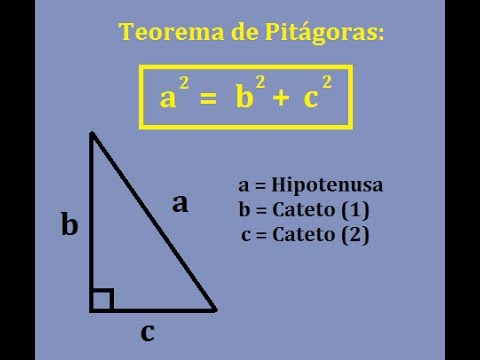# Pythagorean Theorem Proof (Euclid)

TOPICS: Euclid’s Proof     Theorem Proof     Prove Theorem

Euclid was a Greek mathematician and geometrician who lived from 325 to 265 BC and who formulated one of the most famous and simplest proofs about the Pythagorean Theorem.

What Euclid demonstrated was that the area of the square that has the hypotenuse of a right triangle as its side is equal to the sum of the areas that have each of the legs of that same triangle as sides. In the image below we’ll see a graphic demonstration of what we’ve just mentioned. The areas colored in green are equal, and the areas colored in blue are too, meaning that the area of the bottom square is equal to the sum of the areas of the other two squares.

From this, knowing that the area of a square is equal to the square of one of its sides, Euclid was able to deduce that the hypotenuse squared (area of the hypotenuse) is equal to one of the legs squared (area of the green square formed by the first leg) plus the other leg squared (area of the blue square formed by the second leg), and thus derived the formula of the Pythagorean Theorem as we know it today.We also recommend that you see the proof of the Pythagorean Theorem done with water, which we have shown in a previous post and which shows very clearly what Euclid’s proof of the Pythagorean Theorem is based on. You can review it here:

Pythagorean Theorem Proof Using Water